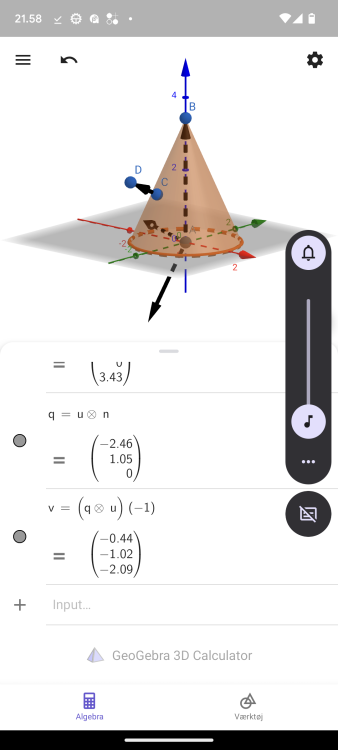# How to calculate edge normal like PolyExtrude

## Recommended Posts

Hello there!

How would you calculate the vector depicted as the red arrows in the image.

PolyExtrude node uses it when given an unshared edge group.

I've tried a polyframe's tangentu and tangent crossed in vex with the Normal. Without luck.

It doesn't seem to be just a continuation of each points inner edges (the not unshared ones.... :/ ). But can't wrap my head around where to go with it.

Thanks

Edited by bastian
##### Share on other sites

Add a pt. wrangle, you will need to compute 2 vectors:

First vector is a cross between v@N and a vector {0,1,0}.
Second vector is a cross between first vector and v@N.
The second vector should be the one you are looking for.

Cheers

•1
##### Share on other sites

@bastian
Prim wrangle (tangent)

```int vtc[];
vtc = primvertices(0, @primnum);

int n = len(vtc);
int jm1, j, jp1;
int pm1, p, pp1;
vector A,B;
for (int i = 0; i < n; i++)
{
jm1 = i - 1;
j = i;
jp1 = i + 1;
if (chi('mode') == 0)
{
jm1 %= (n-1);
jp1 %= (n-1);
} else
{
jm1 = max(0, jm1);
jp1 = min(n-1, jp1);
}
pm1 = vertexpoint(0, vtc[jm1]);
p = vertexpoint(0, vtc[j]);
pp1 = vertexpoint(0, vtc[jp1]);

A = point(0, 'P', p) - point(0, 'P', pm1);
B = point(0, 'P', pp1) - point(0, 'P', p);
setpointattrib(0, 'tangentu', p, normalize(lerp(A, B, 0.5)));
}```

Point wrang-set normal by tangent

```vector up = chv('up');

v@N = normalize(cross(v@tangentu, up));

if (chi('reverse'))
{
v@N *= -1;
}```

•1
##### Share on other sites

Damn, had a hunch Odforce was the place to ask this question.

I'm afk but just gotten done testing @islamooss suggestion on a graphing calculator app I found.

@Librarianserve this up! It's a little more than I have patience testing on the app but I'll test it first thing in the morning!

Thank you!##### Share on other sites

You can try implementing something like Slide Edges to compute the vectors but your topology doesn't seem to have consistent edge loops and rings to make the directions work.

So it depends on how robust you need it vs a one-off.

##### Share on other sites

So I tried your suggestions and both don't work on corners and place where two edges meet, or on edges "facing upwards".
The picture in the post doesn't quite show how inconsistent the mesh is. My mistake.

I've done another pretty "hacky" way of just doing a extra PolyExtrude, wrangle a vector to the extruded edge.
But can imagine it breaking easily with a denser mesh.

I can definitely work with the results here, but would love a consistent method as it isn't the first time I've come across this problem.
Thanks again!

Edited by bastian
comprehension
##### Share on other sites

@bastian Can you post please that mesh.
here is Slide edge + some offset..maybe for someone useful.

##### Share on other sites

Hi,

it seems to work this way:

For each point -> loop over all neighbours where the edge is an open edge. Calculate the cross product of the normal (of incident primitive of the half-edge) and the half-edge direction. Take the average of both normals.

Edited by Aizatulin
•1

## Join the conversation

You can post now and register later. If you have an account, sign in now to post with your account.
Note: Your post will require moderator approval before it will be visible.×   Pasted as rich text.   Paste as plain text instead

Only 75 emoji are allowed.

×   Your previous content has been restored.   Clear editor

×   You cannot paste images directly. Upload or insert images from URL.

×
×